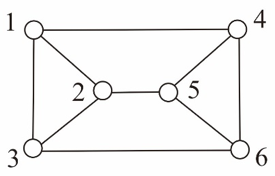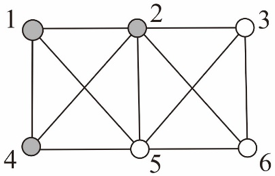시간 제한메모리 제한제출정답맞힌 사람정답 비율
3 초 512 MB94522549.020%

## 문제

Let G be a simple undirected graph with n vertices, whose vertex and edge sets are denoted by V(G) and E(G), respectively. Two edges of G are said to be adjacent if they share a common vertex. Similarly, two vertices of G are said to be adjacent if they share a common edge, in which case the common edge joins the two vertices; an edge and a vertex on that edge are called incident. A subset M of E(G) is called a matching of G if no two edges in M are adjacent; M is called a perfect matching if every vertex of G is incident to exactly one edge of M. So, a matching M of G is perfect if and only if |M| = n/2.

The existence of a perfect matching in G can be decided in polynomial time, thanks to a polynomial-time algorithm for finding a maximum matching, a matching that contains the maximum number of edges. Besides, there are two more interesting problems on the existence of a perfect matching in G:

• Given a partition of V(G) into S and T with |S| = |T| = n/2, does G has a perfect matching in which every edge joins a vertex in S and a vertex in T?
• For every partition of V(G) into S and T with |S| = |T| = n/2, does G has a perfect matching in which every edge joins a vertex in S and a vertex in T?

From the well-known Hall’s marriage theorem, we can derive a condition that characterizes the existence of a required perfect matching for the first question as follows: Let G′ be the spanning subgraph of G with the edges joining vertices both in S or both in T being deleted, i.e., V(G′) = V(G) and E(G′) = {(u,v) ∈ E(G) | either uS and vT or vS and uT. Then, G has a required perfect matching between S and T if and only if G′ has a perfect matching. Moreover, the Hall’s theorem leads to that G′ has a perfect matching if and only if  |N(X)| ≥ |X| for every subset X of S, where N(X) denotes the neighborhood of X, i.e., the set of all vertices in T adjacent to some vertex of X. The question, of course, can be answered in polynomial time, also thanks to a maximum matching algorithm that runs in polynomial time.

Is there an efficient algorithm to answer the second question? A graph that admits a positive answer for the second question is called strongly matchable; that is, a graph G is strongly matchable if G has a perfect matching in which each edge joins two vertices, one in S and the other in T, for every partition of V(G) into S and T with |S| = |T| = n/2. For example, the graph shown in Figure J.1 (a) is strongly matchable because there is a perfect matching for each of the three partitions up to symmetry: M = {(1,4), (2,5), (3,6)} for S = {1,2,3} and T = {4,5,6}; M = {(1,3), (2,5), (4,6)} for S = {1,2,4} and T = {3,5,6}; M = {(1,3), (2,5), (6,4)} for S = {1,2,6} and T = {3,4,5}. However, the graph of (b) is not strongly matchable because there is no perfect matching between S = {1,2,4} and T = {3,5,6}. Your job is to write an efficient running program for deciding whether or not an input graph with an even number of vertices is strongly matchable.(a) (b)

Figure J.1: The graph shown in (a) is strongly matchable, but the graph of (b) is not.

## 입력

Your program is to read from standard input. The first line contains two positive integers n and m, respectively, representing the numbers of vertices and edges of the input graph, where n is even, n ≤ 100, and mn(n-1)/2. It is followed by m lines, each contains two positive integers u and v representing an edge between the vertices u and v of the input graph. It is assumed that the vertices are indexed from 1 to n.

## 출력

Your program is to write to standard output. Print exactly one integer in a line. If the input graph is strongly matchable, the integer should be 1; otherwise, the integer should be -1.

## 예제 입력 1

6 9
1 4
4 6
6 3
3 1
1 2
3 2
4 5
6 5
2 5


## 예제 출력 1

1


## 예제 입력 2

6 11
1 2
2 3
3 6
6 5
5 4
4 1
2 5
1 5
2 4
2 6
3 5


## 예제 출력 2

-1